Home » Quick Introduction to Bag-of-Words (BoW) and TF-IDF for Creating Features from Text

# Quick Introduction to Bag-of-Words (BoW) and TF-IDF for Creating Features from Text

## The Challenge of Making Machines Understand Text

“Language is a wonderful medium of communication”

You and I would have understood that sentence in a fraction of a second. But machines simply cannot process text data in raw form. They need us to break down the text into a numerical format that’s easily readable by the machine (the idea behind Natural Language Processing!).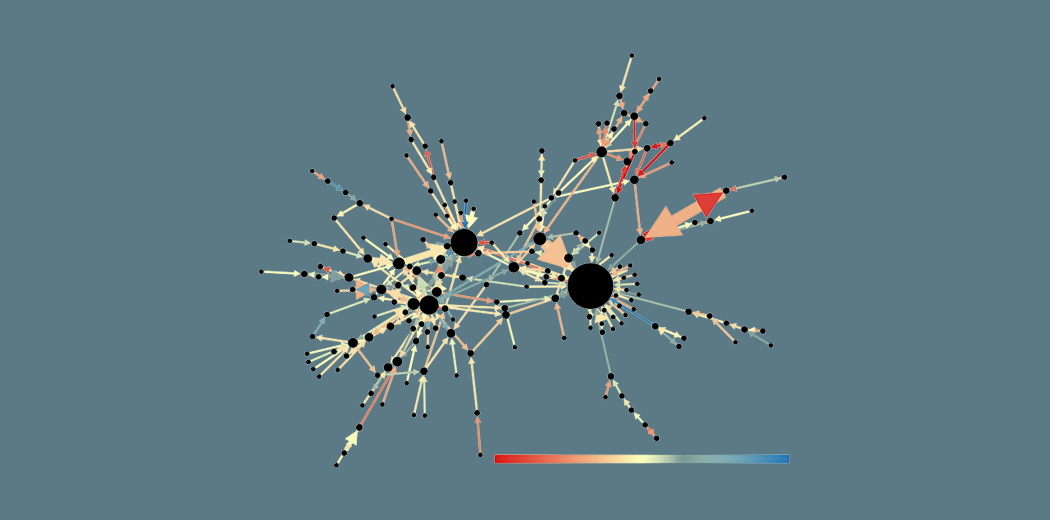This is where the concepts of Bag-of-Words (BoW) and TF-IDF come into play. Both BoW and TF-IDF are techniques that help us convert text sentences into numeric vectors.

I’ll be discussing both Bag-of-Words and TF-IDF in this article. We’ll use an intuitive and general example to understand each concept in detail.

New to Natural Language Processing (NLP)? We’ve got the perfect courses for you to get started:

## Let’s Take an Example to Understand Bag-of-Words (BoW) and TF-IDF

I’ll take a popular example to explain Bag-of-Words (BoW) and TF-DF in this article.

We all love watching movies (to varying degrees). I tend to always look at the reviews of a movie before I commit to watching it. I know a lot of you do the same! So, I’ll use this example here.Here’s a sample of reviews about a particular horror movie:

• Review 1: This movie is very scary and long
• Review 2: This movie is not scary and is slow
• Review 3: This movie is spooky and good

You can see that there are some contrasting reviews about the movie as well as the length and pace of the movie. Imagine looking at a thousand reviews like these. Clearly, there is a lot of interesting insights we can draw from them and build upon them to gauge how well the movie performed.

However, as we saw above, we cannot simply give these sentences to a machine learning model and ask it to tell us whether a review was positive or negative. We need to perform certain text preprocessing steps.

Bag-of-Words and TF-IDF are two examples of how to do this. Let’s understand them in detail.

### Creating Vectors from Text

Can you think of some techniques we could use to vectorize a sentence at the beginning? The basic requirements would be:

1. It should not result in a sparse matrix since sparse matrices result in high computation cost
2. We should be able to retain most of the linguistic information present in the sentence

Word Embedding is one such technique where we can represent the text using vectors. The more popular forms of word embeddings are:

1. BoW, which stands for Bag of Words
2. TF-IDF, which stands for Term Frequency-Inverse Document Frequency

Now, let us see how we can represent the above movie reviews as embeddings and get them ready for a machine learning model.

## Bag of Words (BoW) Model

The Bag of Words (BoW) model is the simplest form of text representation in numbers. Like the term itself, we can represent a sentence as a bag of words vector (a string of numbers).

Let’s recall the three types of movie reviews we saw earlier:

• Review 1: This movie is very scary and long
• Review 2: This movie is not scary and is slow
• Review 3: This movie is spooky and good

We will first build a vocabulary from all the unique words in the above three reviews. The vocabulary consists of these 11 words: ‘This’, ‘movie’, ‘is’, ‘very’, ‘scary’, ‘and’, ‘long’, ‘not’,  ‘slow’, ‘spooky’,  ‘good’.

We can now take each of these words and mark their occurrence in the three movie reviews above with 1s and 0s. This will give us 3 vectors for 3 reviews: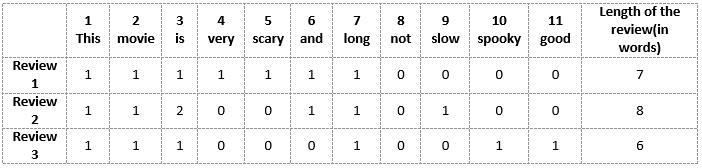Vector of Review 1: [1 1 1 1 1 1 1 0 0 0 0]

Vector of Review 2: [1 1 2 0 0 1 1 0 1 0 0]

Vector of Review 3: [1 1 1 0 0 0 1 0 0 1 1]

And that’s the core idea behind a Bag of Words (BoW) model.

### Drawbacks of using a Bag-of-Words (BoW) Model

In the above example, we can have vectors of length 11. However, we start facing issues when we come across new sentences:

1. If the new sentences contain new words, then our vocabulary size would increase and thereby, the length of the vectors would increase too.
2. Additionally, the vectors would also contain many 0s, thereby resulting in a sparse matrix (which is what we would like to avoid)
3. We are retaining no information on the grammar of the sentences nor on the ordering of the words in the text.

## Term Frequency-Inverse Document Frequency (TF-IDF)

Let’s first put a formal definition around TF-IDF. Here’s how Wikipedia puts it:

“Term frequency–inverse document frequency, is a numerical statistic that is intended to reflect how important a word is to a document in a collection or corpus.”

### Term Frequency (TF)

Let’s first understand Term Frequent (TF). It is a measure of how frequently a term, t, appears in a document, d: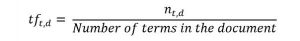Here, in the numerator, n is the number of times the term “t” appears in the document “d”. Thus, each document and term would have its own TF value.

We will again use the same vocabulary we had built in the Bag-of-Words model to show how to calculate the TF for Review #2:

Review 2: This movie is not scary and is slow

Here,

• Vocabulary: ‘This’, ‘movie’, ‘is’, ‘very’, ‘scary’, ‘and’, ‘long’, ‘not’,  ‘slow’, ‘spooky’,  ‘good’
• Number of words in Review 2 = 8
• TF for the word ‘this’ = (number of times ‘this’ appears in review 2)/(number of terms in review 2) = 1/8

Similarly,

• TF(‘movie’) = 1/8
• TF(‘is’) = 2/8 = 1/4
• TF(‘very’) = 0/8 = 0
• TF(‘scary’) = 1/8
• TF(‘and’) = 1/8
• TF(‘long’) = 0/8 = 0
• TF(‘not’) = 1/8
• TF(‘slow’) = 1/8
• TF( ‘spooky’) = 0/8 = 0
• TF(‘good’) = 0/8 = 0

We can calculate the term frequencies for all the terms and all the reviews in this manner: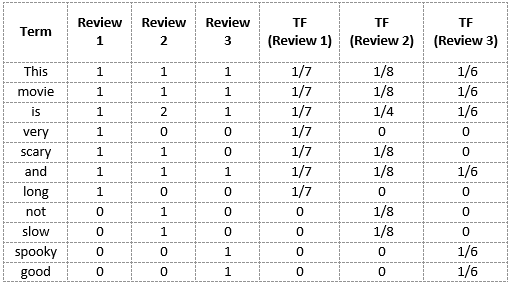### Inverse Document Frequency (IDF)

IDF is a measure of how important a term is. We need the IDF value because computing just the TF alone is not sufficient to understand the importance of words: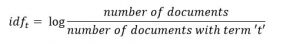We can calculate the IDF values for the all the words in Review 2:

IDF(‘this’) =  log(number of documents/number of documents containing the word ‘this’) = log(3/3) = log(1) = 0

Similarly,

• IDF(‘movie’, ) = log(3/3) = 0
• IDF(‘is’) = log(3/3) = 0
• IDF(‘not’) = log(3/1) = log(3) = 0.48
• IDF(‘scary’) = log(3/2) = 0.18
• IDF(‘and’) = log(3/3) = 0
• IDF(‘slow’) = log(3/1) = 0.48

We can calculate the IDF values for each word like this. Thus, the IDF values for the entire vocabulary would be: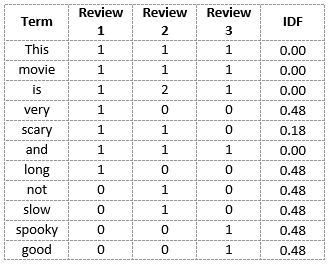Hence, we see that words like “is”, “this”, “and”, etc., are reduced to 0 and have little importance; while words like “scary”, “long”, “good”, etc. are words with more importance and thus have a higher value.

We can now compute the TF-IDF score for each word in the corpus. Words with a higher score are more important, and those with a lower score are less important: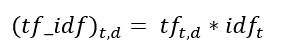We can now calculate the TF-IDF score for every word in Review 2:

TF-IDF(‘this’, Review 2) = TF(‘this’, Review 2) * IDF(‘this’) = 1/8 * 0 = 0

Similarly,

• TF-IDF(‘movie’, Review 2) = 1/8 * 0 = 0
• TF-IDF(‘is’, Review 2) = 1/4 * 0 = 0
• TF-IDF(‘not’, Review 2) = 1/8 * 0.48 = 0.06
• TF-IDF(‘scary’, Review 2) = 1/8 * 0.18 = 0.023
• TF-IDF(‘and’, Review 2) = 1/8 * 0 = 0
• TF-IDF(‘slow’, Review 2) = 1/8 * 0.48 = 0.06

Similarly, we can calculate the TF-IDF scores for all the words with respect to all the reviews: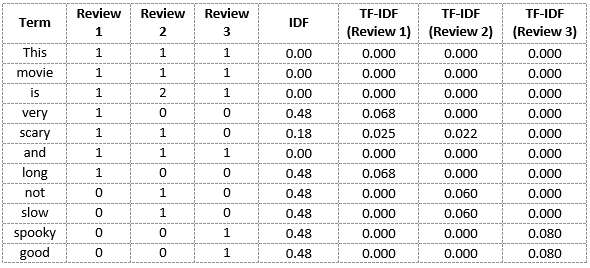We have now obtained the TF-IDF scores for our vocabulary. TF-IDF also gives larger values for less frequent words and is high when both IDF and TF values are high i.e the word is rare in all the documents combined but frequent in a single document.

## End Notes

Let me summarize what we’ve covered in the article:

1. Bag of Words just creates a set of vectors containing the count of word occurrences in the document (reviews), while the TF-IDF model contains information on the more important words and the less important ones as well.
2. Bag of Words vectors are easy to interpret. However, TF-IDF usually performs better in machine learning models.

While both Bag-of-Words and TF-IDF have been popular in their own regard, there still remained a void where understanding the context of words was concerned. Detecting the similarity between the words ‘spooky’ and ‘scary’, or translating our given documents into another language, requires a lot more information on the documents.

This is where Word Embedding techniques such as Word2Vec, Continuous Bag of Words (CBOW), Skipgram, etc. come in. You can find a detailed guide to such techniques here:

You can also read this article on our Mobile APP